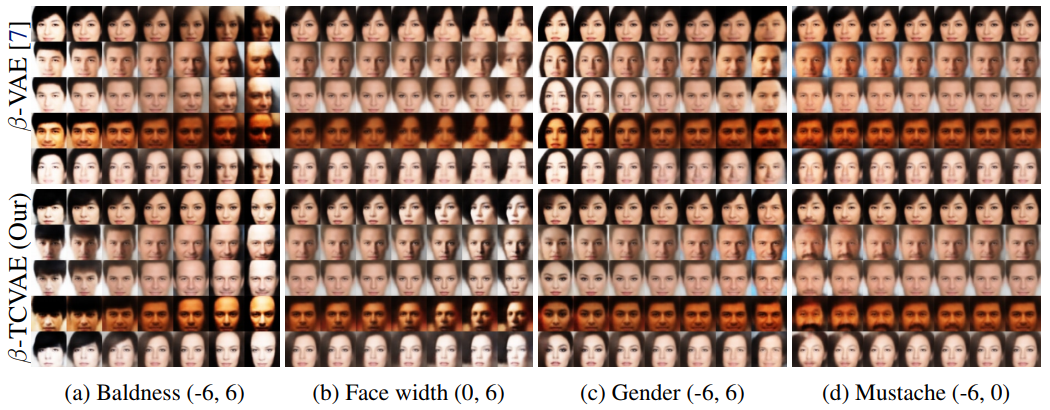WHY?

$\beta$-VAE is known to disentangle latent variables.

WHAT?

This paper further decomposed the KL divergence term in b-VAE (TC-Decomposition).

The first term of the right hand side is referred to as the index-code mutual information(MI) which is the mutual information between data and latent variable. The second term is referred to as the total correlation(TC) which measure statistical dependency between variables. The third term is dimension-wise KL which is complexity penalty from diagonal Gaussian. Disentangling is mostly affected by the second term(TC) but is is hard to decompose due to q(z).
Evaluation of $q(z) = \mathbb{E}_{p(N)}[q(z|n)]$ require statistics of entire dataset. Instead of getting statistics of the dataset, that of mini-batch can be use to approximate it.

Therefore, the objective function of $\beta$-TCVAE is as follows.

Increasing the beta while fixing alpha and gamma to 1 leads to better disentanglement.

So?According to disentanglement measure(Mutual Information Gap:MIG) this paper suggested, beta-TCVAE showed better performance than beta-vae.

Critic

Great improvement with simple modification and clean theoratical backup.

Chen, Tian Qi, et al. “Isolating Sources of Disentanglement in Variational Autoencoders.” arXiv preprint arXiv:1802.04942 (2018).